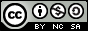- 目录 -
LeetCode 214 最短回文串(kmp、next应用)

输入: "aacecaaa"



输入: "abcd"



## 思路

kmp

• next[i] 代表 从 [0,i-1] 的最长的相同真前后缀的长度

## 代码

  1 2 3 4 5 6 7 8 9 10 11 12 13 14 15 16 17 18 19 20 21 22 23 24 25 26  class Solution { public: vector nxt; void get_next(string &p) { int len = p.length(); int j = 0, k = -1; nxt = -1; while (j < len) if (k == -1 || p[j] == p[k]) nxt[++j] = ++k; else k = nxt[k]; } string shortestPalindrome(string s) { string rs = s; reverse(rs.begin(), rs.end()); string ns = s + "#" + rs; int n = ns.size(); nxt.resize(n + 1); get_next(ns); return rs.substr(0, rs.size() - nxt[n]) + s; } };